## RD Sharma Class 8 Solutions Chapter 1 Rational Numbers Ex 1.8

These Solutions are part of RD Sharma Class 8 Solutions. Here we have given RD Sharma Class 8 Solutions Chapter 1 Rational Numbers Ex 1.8

Other Exercises

Question 1.
Find a rational number between -3 and 1.
Solution: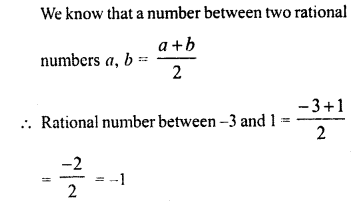Question 2.
Find any five rational number less than 1.
Solution:Question 3.
Find four rational numbers between $$\frac { -2 }{ 9 }$$ and $$\frac { 5 }{ 9 }$$ .
Solution:Question 4.
Find two rational numbers between $$\frac { 1 }{ 5 }$$ and $$\frac { 1 }{ 2 }$$ .
Solution: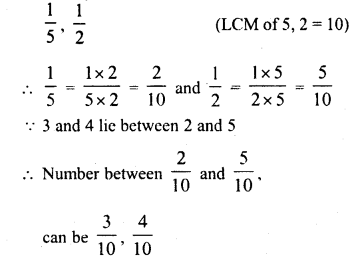Question 5.
Find ten rational numbers between $$\frac { 1 }{ 4 }$$ and $$\frac { 1 }{ 2 }$$ .
Solution: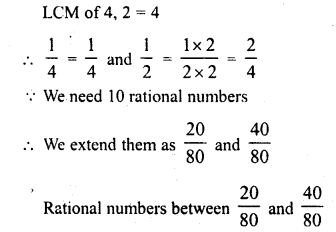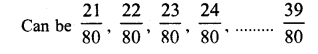Question 6.
Find ten rational numbers between $$\frac { -2 }{ 5 }$$ and $$\frac { 1 }{ 2 }$$ .
Solution: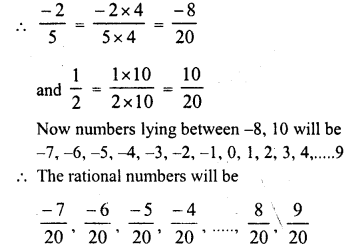Question 7.
Find ten rational numbers between $$\frac { 3 }{ 5 }$$ and $$\frac { 3 }{ 4 }$$ .
Solution: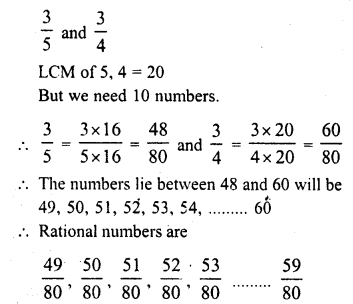Hope given RD Sharma Class 8 Solutions Chapter 1 Rational Numbers Ex 1.8 are helpful to complete your math homework.

If you have any doubts, please comment below. Learn Insta try to provide online math tutoring for you.